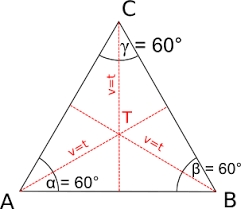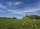# Three parallels

The vertices of an equilateral triangle lie on 3 different parallel lines. The middle line is 5 m and 3 m distant from the end lines. Calculate the height of this triangle.

Result

v =  7 m

#### Solution:

$a^2 = 3^2 +x^2 \ \\ a^2 = 5^2 + y^2 \ \\ a^2 = 8^2 + (y-x)^2 \ \\ \ \\ 3^2 +x^2 = 5^2 + y^2 \ \\ 3^2 +x^2 = 8^2 + (y-x)^2 \ \\ \ \\ 3^2 +x^2 = 8^2 + y^2-2xy +x^2 \ \\ 3^2 = 8^2 + y^2-2xy \ \\ \ \\ x = (8^2 - 3^2 + y^2) / (2y) \ \\ \ \\ y = 11 / \sqrt{ 3 } \doteq 6.3509 \ m \ \\ \ \\ x = (8^2 - 3^2 + y^2) / (2y) = (8^2 - 3^2 + 6.3509^2) / (2 \cdot \ 6.3509) \doteq 7.5056 \ m \ \\ \ \\ a = \sqrt{ 3^2 + x^2 } = \sqrt{ 3^2 + 7.5056^2 } \doteq 8.0829 \ m \ \\ \ \\ v^2 = a^2 - (a/2)^2 \ \\ \ \\ v = \sqrt{ a^2 - (a/2)^2 } = \sqrt{ 8.0829^2 - (8.0829/2)^2 } = 7 = 7 \ \text { m }$Our examples were largely sent or created by pupils and students themselves. Therefore, we would be pleased if you could send us any errors you found, spelling mistakes, or rephasing the example. Thank you!

Leave us a comment of this math problem and its solution (i.e. if it is still somewhat unclear...):Be the first to comment!#### Following knowledge from mathematics are needed to solve this word math problem:

Looking for help with calculating roots of a quadratic equation? Do you have a linear equation or system of equations and looking for its solution? Or do you have quadratic equation? Do you want to convert length units? Pythagorean theorem is the base for the right triangle calculator. See also our trigonometric triangle calculator.

## Next similar math problems:

1. Sphere from tree pointsEquation of sphere with three point (a,0,0), (0, a,0), (0,0, a) and center lies on plane x+y+z=a
2. Theorem proveWe want to prove the sentence: If the natural number n is divisible by six, then n is divisible by three. From what assumption we started?
3. CathetiThe hypotenuse of a right triangle is 41 and the sum of legs is 49. Calculate the length of its legs.
4. RTriangle 17The hypotenuse of a right triangle is 17 cm. If you decrease both two legs by 3 cm you will reduce the hypotenuse by 4 cm. Determine the length of this legs.
5. Triangle ABCTriangle ABC has side lengths m-1, m-2, m-3. What has to be m to be triangle a) rectangular b) acute-angled?
6. AngleDetermine the size of the smallest internal angle of a right triangle which angles forming the successive members of the arithmetic sequence.
7. Right angled triangleHypotenuse of a right triangle is 17 cm long. When we decrease length of legs by 3 cm then decrease its hypotenuse by 4 cm. Determine the size of legs.
8. Distance problem 2A=(x,2x) B=(2x,1) Distance AB=√2, find value of xFind the roots of the quadratic equation: 3x2-4x + (-4) = 0.
10. RootsDetermine the quadratic equation absolute coefficient q, that the equation has a real double root and the root x calculate: ?
11. Linsys2Solve two equations with two unknowns: 400x+120y=147.2 350x+200y=144
12. VariableFind variable P: PP plus P x P plus P = 160
13. Ball gameRichard, Denis and Denise together scored 932 goals. Denis scored 4 goals over Denise but Denis scored 24 goals less than Richard. Determine the number of goals for each player.
14. Men, women and childrenOn the trip went men, women and children in the ratio 2:3:5 by bus. Children pay 60 crowns and adults 150. How many women were on the bus when a bus was paid 4,200 crowns?
15. EquationEquation ? has one root x1 = 8. Determine the coefficient b and the second root x2.
16. DiscriminantDetermine the discriminant of the equation: ?
17. Solve 3Solve quadratic equation: (6n+1) (4n-1) = 3n2